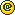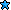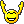SB Physics Help Room Sucks!
Would you like to react to this message? Create an account in a few clicks or log in to continue.

# Ch 24-3 Quiz##Ch 24-3 Quiz

Question 1: ((ln(factor))/(decay constant))/31536000

The number in the denominator is the amount of seconds in a year.

helping
Guest

##Re: Ch 24-3 Quiz

Question 2:

decays per day/(e^-("thousand years ago value"*365000*(.69315/(half life * 365))))

helping
Guest

##Re: Ch 24-3 Quiz

Question 3:

(9*10^9*(# of protons)*(1.6*10^-19)^2)/(distance)

Question 4:

((9*10^9*2*(# of protons)*(1.6*10^-19)^2)/distance)/(1.6*10^-13)

Good luck!

helping
Guest

##Re: Ch 24-3 Quiz

for number 4 y did you divide by 1.6E-13

hey
Guest

##Re: Ch 24-3 Quiz

To convert to MeV, you multiply 10^6 to your usual 10^-19.

Helping
Guest

##4

I am getting # 4 WRONG

any help

4
Guest

##Re: Ch 24-3 Quiz

Number 4 is exactly like number 3 except that you multiply by two in the numerator and then divide the whole answer by 1.6E-13.

helping
Guest

##4

thanx a lot i got it nowa
Guest

##Question 2

I am having problems figuring out number 2. I am using the formula provided above but it doesn't seem to work for me.

The half life of radioactive C14 nuclei is 5730 years. If the activity of a sample today is 24.61 decays per day, what was its activity 2.426 thousand years ago (in decays per day) (sheet 23,23') ? Indicate with a negative (positive) sign whether the number of radioactive nuclei as a function of time obeys the same (a different) exponential decay law as the activity as a function of time.

24.61/(e^(2.426*365000*9.69315/(5730*365)))) and I get my answer as -18.3510....what am i doing wrong?

Guest786
Guest

##question 2 correction

this is what i did

24.61/(e^(2.426*365000*(.69315/(5730*365)))) and I get my answer as -18.3510....what am i doing wrong?

Guest786
Guest

##Re: Ch 24-3 Quiz

this is what i did

24.61/(e^(2.426*365000*(.69315/(5730*365)))) and I get my answer as -18.3510....what am i doing wrong?

ur missing a (-) sign after e^
it should be 24.61/(e^-(2.426*365000*(.69315/(5730*365))))

guest123
Guest

##Number 2

Hey guys, I got the other 3 but for some strange reason im stuck on number 2. I tried to follow all the appropriate steps but keep getting an incorrect answer on mapleTA. This is my question...
2) The half life of radioactive C14 nuclei is 5730 years. If the activity of a sample today is 20.85 decays per day, what was its activity 8.613 thousand years ago (in decays per day) (sheet 23,23') ? Indicate with a negative (positive) sign whether the number of radioactive nuclei as a function of time obeys the same (a different) exponential decay law as the activity as a function of time

This is what i did...
20.85/ (e^-(8.163 * 365000 * (.69315/ (5730* 365))))= 55.97
mapleTA says im wrong. Maybe one of you guys can help me.
Obviously im making a retarded mistake somewhere and I cant seem to figure out where lol so pleaseee. Will be eternally grateful!!!Money23
Guest

##n/m

Nevermind guys i got it!!! finally...Money23
Guest

##Re: Ch 24-3 Quiz

Can you explain number one please?

asda
Guest

##Re: Ch 24-3 Quiz

ditto, can someone please explain the mathematical formulation that brings one to the answer for number one?

zZtoP
Guest

##Re: Ch 24-3 Quiz

You use the formula on Sheet 23 N(t)=Noe^-lambdat
divide No over so you get N/No which is the same thing as the factor of decrease or increase.
N/No = e^-lamdat
ln(N/No) = lamdat
(ln(N/No)/lamda) = t
and once you solve for t you gotta convert it to years which is the big number you divide by.

PhyPhy
Guest

##Re: Ch 24-3 Quiz